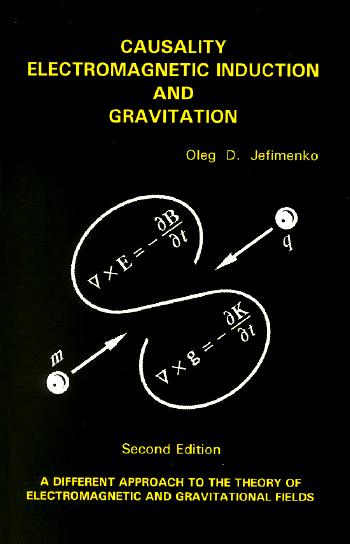# Causality, Electromagnetic Induction and Gravitation

### Hardback - List Price US\$ 32.50 ISBN 0-917406-22-2

Hardback is sold out. Please order paperback listed above.This book is a strikingly new exploration of the fundamentals of Maxwell's electromagnetic theory and of Newton's theory of gravitation. Starting with an analysis of causality in the phenomenon of electromagnetic induction, the author discovers a series of heretofore unknown or overlooked electromagnetic interdependencies and equations. One of the most notable new results is the discovery that Maxwell's equations do not depict cause and effect relations between electromagnetic phenomena: causal dependencies in electromagnetic phenomena are found to be described by solutions of Maxwell's equations in the form of retarded electric and magnetic field integrals. A consequence of this discovery is that, contrary to the generally accepted view, time-variable electric and magnetic fields cannot cause each other and that both fields are simultaneously created by their true causative sources -- time-dependent electric charges and currents. Another similarly important discovery is that Lenz's law of electromagnetic induction is a manifestation of the previously ignored electric force produced by the time-dependent electric currents. These discoveries lead to important new methods of calculations of various electromagnetic effects in time- depended electromagnetic systems. The new methods are demonstrated by a variety of illustrative examples. Continuing his analysis of causal electromagnetic relations, the author finds that these relations are closely associated with the law of momentum conservation, and that with the help of the law of momentum conservation one can analyze causal relations not only in electromagnetic but also in gravitational systems. This leads to the discovery that in the time-dependent gravitational systems the momentum cannot be conserved without a second gravitational force field, which the author calls the "cogravitational, or Heaviside's, field." This second field, first predicted by Heaviside, relates to the gravitational field proper just as the magnetic field relates to the electric field. The author then generalizes Newton's gravitational theory to time-dependent systems and derives causal gravitational equations in the form of two retarded integrals similar to the retarded integrals for the electric and magnetic fields introduced previously. One of the most important consequences of the causal gravitational equations is that a gravitational interaction between two bodies involves not one force (as in Newton's theory) but as many as five different forces corresponding to the five terms in the two retarded gravitational and cogravitational field integrals. These forces depend not only on the masses and separation of the interacting bodies, but also on their velocity and acceleration and even on the rate of change of their masses. A series of illustrative examples on the calculation of these new forces is provided and a graphical representation of these forces is given. The book concludes with a discussion of the possibility of antigravitation as a consequence of the negative equivalent mass of the gravitational field energy. The book is written in the style and format of a textbook. The clear presentation, the detailed derivations of all the basic formulas and equations, and the many illustrative examples make this book well suitable not only for independent studies but also as a supplementary textbook in courses on electromagnetic theory and gravitation. The second edition of the book refines and improves the first edition, especially in the presentation and development of Newton's gravitational theory generalized to time-dependent gravitational systems. The book has been augmented by several new Appendixes. Particularly notable are Appendixes 5, 6, and 8. Appendixes 5 and 6 present novel "dynamic" electric and gravitational field maps of rapidly moving charges and masses. Appendix 8 contains the little-known but extremely important Heaviside's 1893 article on the generalization of Newton's gravitational theory.